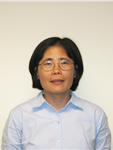# Dr. Fuxia Cheng

Professor
MathematicsOffice
Stevenson Hall - STV 305
• Education
• Research

### Current Courses

350.001Applied Probability Models

353.001Regression And Time Series Analysis

351.001Statistics And Data Analysis

351.001Statistics And Data Analysis

351.002Statistics And Data Analysis

458.001The Design Of Experiments

### Journal Article

Cheng, F. The Lp consistency of error density estimator in censored linear regression. COMMUNICATIONS IN STATISTICS—THEORY AND METHODS (2019)
Cheng, F. Glivenko-Cantelli Theorem for Kernel Error Distribution Estimator in the First-order Autoregressive Model. Statistics & Probability Letters 139 (2018): 95-102.
Sun, S., Cheng, F., & . Bootstrapping the Expected Shortfall. Theoretical Economics Letters, 2018, 8, 685-698 (2018)
Cheng, F. Strong Convergence of the distribution estimator in the nonlinear autoregressive time series.. Journal of Multivariate Analysis 142 (2015): 41-47.
Cheng, F. Maximum Deviation of Error Density Estimators in Censored Linear Regression. Statistics & Probability Letters 82 (2012): 1657-1664.

### Manuscript

Cheng, F., Sun, S., & Wen, M. Asymptotics for L2-norm of ARCH Innovation Density Estimator. Journal of Statistical Planning and Inference 141 (2012): 3771-3779.
Cheng, F., & Yang, L. Strong Uniform Consistency Rates of Kernel Estimators of Cumulative Distribution Functions. Statistics & Probability Letters (2012)
Cheng, F., & Wen, M. The L1 strong consistency of ARCH innovation density estimator. Statistics & Probability Letters 81 (2011): 548-551.
Cheng, F. Variance Estimation in Nonlinear Autoregressive Time Series Models. Journal of Statistical Planning and Inference 141 (2011): 1588-1592.
Cheng, F., Huang, H., & Zhou, H. Gaussian Process Based Bayesian Semiparametric Quantitative Trait Loci Interval Mapping. Biometrics 66 (2010): 222-232.

### Presentations

Asymptotics of Distribution Estimation in Linear Autoregressive Models. the International Conference on Statistics, Mathematical Modelling and Analysis (SMMA 2018) September 15-17, 2018 in Chongqin, China. (2018)
Asymptotic properties of density estimators in censored linear regression. International Workshop on Modern Methods in Financial Statistics. Soochow University. (2012)
Density Estimators in Censored Linear Regression. Department of Mathematics. Illinois State University. (2011)
Density and Variance Estimation in Nonlinear Autoregressive Time Series. oral presentation. First Wuxi International Statistics Forum. (2011)
Nonparametric estimation of conditional VaR and expected shortfall. Department of Mathematics. Illinois State University. (2011)
Asymptotic properties in ARCH(p)-time series. Institute of Statistical Science of Academic Sinica. (2010)
Asymptotic properties in ARCH(p)-time series. Department of Statistics at National Cheng Kung University. (2010)
"Global Property of Error Density Estimation in Nonlinear Autoregressive Time Series Models. ICSA 2010 Applied Statistics Symposium. (2010)